mscroggs.co.uk
mscroggs.co.uksubscribe

Blog

2017-03-27
Tomorrow, the new 12-sided one pound coin is released.
Although I'm excited about meeting this new coin, I am also a little sad, as its release ends the era in which all British coins are shapes of constant width.

Shapes of constant width

A shape of constant width is a shape that is the same width in every direction, so these shapes can roll without changing height. The most obvious such shape is a circle. But there are others, including the shape of the seven-sided 50p coin.
As shown below, each side of a 50p is part of a circle centred around the opposite corner. As a 50p rolls, its height is always the distance between one of the corners and the side opposite, or in other words the radius of this circle. As these circles are all the same size, the 50p is a shape of constant width.
Shapes of constant width can be created from any regular polygon with an odd number of sides, by replacing the sides by parts of circles centred at the opposite corner. The first few are shown below.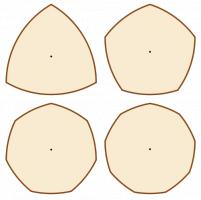en wiki user LEMeZza, CC BY-SA 3.0
It's also possible to create shapes of constant width from irregular polygons with an odd number, but it's not possible to create them from polygons with an even number of sides. Therefore, the new 12-sided pound coin will be the first non-constant width British coin since the (also 12-sided) threepenny bit was phased out in 1971.
Back in 2014, I wrote to my MP in an attempt to find out why the new coin was not of a constant width. He forwarded my letter to the Treasury, but I never heard back from them.

Pizza cutting

When cutting a pizza into equal shaped pieces, the usual approach is to cut along a few diameters to make triangles. There are other ways to fairly share pizza, including the following (that has appeared here before as an answer to this puzzle):
The slices in this solution are closely related to a triangle of constant width. Solutions can be made using other shapes of constant width, including the following, made using a constant width pentagon and heptagon (50p):
There are many more ways to cut a pizza into equal pieces. You can find them in Infinite families of monohedral disk tilings by Joel Haddley and Stephen Worsley .
You can't use the shape of a new pound coin to cut a pizza though.
Edit: Speaking of new £1 coins, I made this stupid video with Adam "Frownsend" Townsend about them earlier today:

References

Infinite families of monohedral disk tilings by Joel Haddley and Stephen Worsley. December 2015. [link]

Similar posts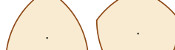New machine unfriendly £1 coin, pt. 2New machine unfriendly £1 coin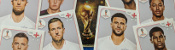World Cup stickers 2018, pt. 3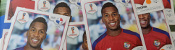World Cup stickers 2018, pt. 2

Comments in green were written by me. Comments in blue were not written by me.

Allowed HTML tags: <br> <a> <small> <b> <i> <s> <sup> <sub> <u> <spoiler> <ul> <ol> <li>
To prove you are not a spam bot, please type "prime" in the box below (case sensitive):
2017-02-25
Recently, I've noticed a few great examples of misleading uses of numbers in news articles.
On 15 Feb, BBC News published a breaking news article with the headline "UK unemployment falls by 7,000 to 1.6m". This fall of 7,000 sounds big; but when compared to the total of 1.6m, it is insignificant. The change could more accurately be described as a fall from 1.6m to 1.6m.
But there is a greater problem with this figure. In the original Office of National Statistics (ONS) report, the fall of 7,000 was accompanied by a 95% confidence interval of ±80,000. When calculating figures about large populations (such as unemployment levels), it is impossible to ask every person in the UK whether they are employed or not. Instead, data is gathered from a sample and this is used to estimate the total number. The 95% confidence interval gives an idea of the accuracy of this estimation: 95% of the time, the true number will lie of the confidence interval. Therefore, we can think of the 95% confidence interval as being a range in which the figure lies (although this is not true, it is a helpful way to think about it).
Compared to the size of its confidence interval (±80,000), the fall of 7,000 is almost indistinguishable from zero. This means that it cannot be said with any confidence whether the unemployment level rose or fell. This is demonstrated in the following diagram.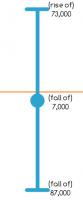A fall of 7,000 ± 80,000. The orange line shows no change.
To be fair to the BBC, the headline of the article changed to "UK wage growth outpaces inflation" once the article was upgraded from breaking news to a complete article, and a mention of the lack of confidence in the change was added.
On 23 Feb, I noticed another BBC News with misleading figures: Net migration to UK falls by 49,000. This 49,000 is the difference between 322,000 (net migration for the year ending 2015) and 273,000 (net migration for the year ending 2016). However both these figures are estimates: in the original ONS report, they were placed in 95% confidence intervals of ±37,000 and ±41,000 respectively. As can be seen in the diagram below, there is a significant portion where these intervals overlap, so it cannot be said with any confidence whether or not net immigration actually fell.
Perhaps the blame for this questionable figure lies with the ONS, as it appeared prominently in their report while the discussion of its accuracy was fairly well hidden. Although I can't shift all blame from the journalists: they should really be investigating the quality of these figures, however well advertised their accuracy is.
Both articles criticised here appeared on BBC News. This is not due to the BBC being especially bad with figures, but simply due to the fact that I spend more time reading news on the BBC than in other places, so noticed these figures there. I quick Google search reveals that the unemployment figure was also reported, with little to no discussion of accuracy, by The Guardian, the Financial Times, and Sky News.

Similar postsWorld Cup stickers 2018, pt. 3World Cup stickers 2018, pt. 2A bad Puzzle for TodayA 20,000-to-1 baby?

Comments in green were written by me. Comments in blue were not written by me.
2017-12-26
I've seen archaeologists claiming proof that event A happened before event B because the radiocarbon date of A was 50 years before B. Except the standard error on both dates was 100 years. They even showed the error bars in their own graphics, but seemed to not understand what it meant.

My favorite species of ignoring the measurement error is the metric conversion taken to way too many decimal places. The hike was 50 miles (80.467 kilometers) long.

Allowed HTML tags: <br> <a> <small> <b> <i> <s> <sup> <sub> <u> <spoiler> <ul> <ol> <li>
To prove you are not a spam bot, please type "d" then "e" then "c" then "a" then "g" then "o" then "n" in the box below (case sensitive):
2016-05-04
Back in 2014, I calculated the expected cost of filling Panini world cup sticker album. I found that you should expect to buy 4505 stickers, or 1285 if you order the last 100 from the Panini website (this includes the last 100). This would cost £413.24 or £133.99 respectively.
Euro 16 is getting close, so it's sticker time again. For the Euro 16 album there are 680 stickers to collect, 40 more than 2014's 640 stickers. Using the same calculation method as before, to fill the Euro 16 album, you should expect to buy 4828 stickers (£442.72), or 1400 (£134.32) if you order the last 100.
This, however, does not tell the whole story. Anyone who has collected stickers as a child or an adult will know that half the fun comes from swapping your doubles with friends. Getting stickers this way is not taken into account in the above numbers.

Simulating a sticker collection

Including swaps makes the situation more complicated: too complicated to easily calculate the expected cost of a full album. Instead, a different method is needed. The cost of filling an album can be estimated by simulating the collection lots of times and taking the average of the cost of filling the album in each simulation. With enough simulations, this estimate will be very close the the expected cost.
To get an accurate estimation, simulations are run, calculating the running average as they go, until the running averages after recent simulations are close together. (In the examples, I look for the four most recent running averages to be within 0.01.) The plot below shows how the running average changes as more simulations are performed.
The simulations estimate the number of stickers needed as 4500. This is very close to the 4505 I calculated last year.
Now that the simulations are set up, they can be used to see what happens if you have friends to swap with.

What should I do?

The plots below shows how the number of stickers you need to buy each changes based on how many friends you have.Stickers needed if you and your friends order no stickers.Stickers needed if you and your friends all order the last 100 stickers. The last 100 are not counted.
In both these cases, having friends reduces the number of stickers you need to buy significantly, with your first few friends making the most difference.
Ordering the last 100 stickers looks to be a better idea than ordering no stickers. But how many stickers should you order to minimise the cost? When you order stickers, you are guaranteed to get those that you need, but they cost more: ordered stickers cost 14p each, while stickers in 6 pack multipacks come out at just 9.2p each. The next plot shows how the cost changes based on how many you order.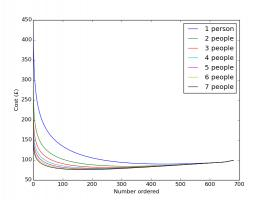The expected cost of filling an album based on number of people in group and number of stickers ordered.
Each of the coloured curves represents a group of a different size. For each group, ordering no stickers works out the most expensive—this is expected as so many stickers must be bought to find the last few stickers—and ordering all the stickers also works out as not the best option. The best number to order is somewhere in the middle, where the curve reaches its lowest point. The minimum points on each of these curves are summarised in the next plots:How the number you should order changes with the number of people in the group.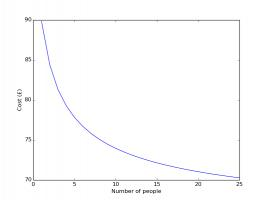How the cost changes with the number of people in the group.
Again, having friends to swap with dramatically reduces the cost of filling an album. In fact, it will almost definitely pay off in future swaps if you go out right now and buy starter packs for all your friends...

Similar postsWorld Cup stickers 2018, pt. 3World Cup stickers 2018, pt. 2World Cup stickers 2018World Cup stickers

Comments in green were written by me. Comments in blue were not written by me.

Allowed HTML tags: <br> <a> <small> <b> <i> <s> <sup> <sub> <u> <spoiler> <ul> <ol> <li>
To prove you are not a spam bot, please type "sixa-y" backwards in the box below (case sensitive):
2015-10-21
This post also appeared on the Chalkdust Magazine blog.
If you're like me, then you will be disappointed that all of the home nations have been knocked out of the Rugby World Cup. If you're really like me, doing some maths related to rugby will cheer you up...
The scoring system in rugby awards points in packets of 3, 5 and 7. This leads a number of interesting questions that you can find in my guest puzzle on Alex Bellos's Guardian blog. In this blog post, we will focus on another area of rugby: conversion kicking.

Conversion kicks

When a try is scored by putting the ball down behind the line, the scoring team gets to take a conversion kick. This kick must be taken in line with where the try was scored but it is up to the kicker how far away the kick should be taken. But how far back should the ball be taken to make the kick easiest?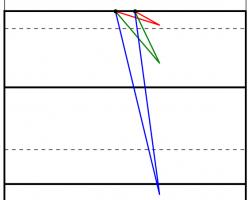Too close (red) and too far away (blue) will give small angles to aim at. Somewhere in the middle is needed (green).
One way to answer this question is to look to maximise the angle between the posts which the kicker will have to aim at: if the kick is taken too close to or too far from the goal line there will be a very thin angle to aim at. Somewhere between these extremes there will be a maximum angle to aim at.
When looking to maximise this angle, we can use one of the 'circle theorems' which have tormented many generations of GCSE maths students: 'angles subtended by the same arc at the circumference are equal'. This means that if a circle is drawn going through both posts, then the angle made at any point on this circle will be the same.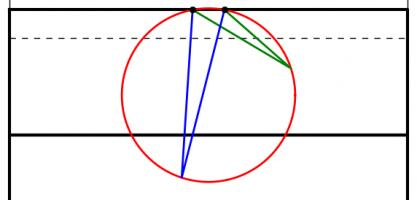The angles made by the red and blue lines are equal because 'angles subtended by the same arc at the circumference are equal'.
A larger circle drawn through the posts will give a smaller angle. If a vertical line is drawn which just touches the right of the circle, then the point at which it touches the circle will be the best place on this line to take a kick. This is because any other point on the line will be on a larger circle and so make a smaller angle.
Using this method for circles of different sizes leads to the following diagram, which shows where the kick should be taken for every position a try could be scored:
This, however, is not the best place to take the kick.

Taking account of height

When a try is scored near the posts, the above method recommends a position from where the ball must be kicked at an impossibly steep angle to go over. To deal with this problem, we are going to have to look at the situation from the side.
When kicked, the ball will travel along a parabola (ignoring air resistance and wind as their effects will be small[citation needed]). Given a distance from the posts, there will be two angles which the ball can be kicked at and just make it over the bar. Kicking at any angle between these two will lead to a successful conversion. Again, we have an angle which we would like to maximise.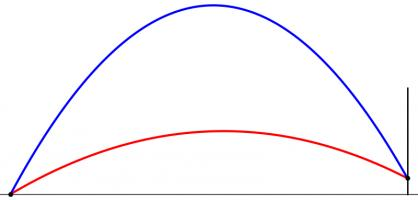The highest (blue) and lowest (red) the ball can be kicked while still going over the bar.
However, the position where this angle is maximised is very unlikely to also maximise the angle we looked at earlier. To find the best place to kick from, we need to find a compromise point where both angles are quite big.
To do this, imagine that the kicker is standing inside a large sphere. For each point on the sphere, kicking the ball at the point will either lead to it going over or missing. We can draw a shape on the sphere so that aiming inside the shape will lead to scoring. Our sensible kicker will aim at the centre of this shape.
But our kicker will not be able to aim perfectly: there will be some random variation. We can predict that this variation will follow a Kent distribution, which is like a normal distribution but on the surface of a sphere. We can use this distribution to calculate the probability that our kicker will score. We would like to maximise this probability.
The Kent distribution can be adjusted to reflect the accuracy of the kicker. Below are the optimal kicking positions for an inaccurate, an average and a very accurate kicker.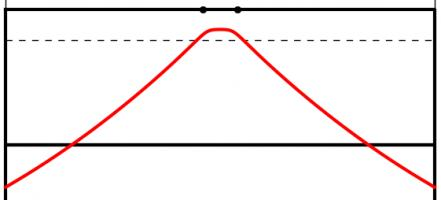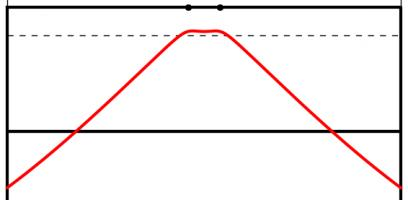The best place to take a kick for a bad kicker (top), an average kicker (middle) and a good kicker (bottom). All the kickers kick the ball at 30m/s.
As you might expect, the less accurate kicker should stand slightly further forwards to make it easier to aim. Perhaps surprisingly, the good kicker should stand further back when between the posts than when in line with the posts.
The model used to create these results could be further refined. Random variation in the speed of the kick could be introduced. Or the kick could be made to have more variation horizontally than vertically: there are parameters in the Kent distribution which allow this to be easily adjusted. In fact, data from players could be used to determine the best position for each player to kick from.
In addition to analysing conversions, this method could be used to determine the probability of scoring 3 points from any point on the pitch. This could be used in conjunction with the probability of scoring a try from a line-out to decide whether kicking a penalty for the posts or into touch is likely to lead to the most points.
Although estimating the probability of scoring from a line-out is a difficult task. Perhaps this will give you something to think about during the remaining matches of the tournament.

Similar postsWorld Cup stickers 2018, pt. 3World Cup stickers 2018, pt. 2World Cup stickers 2018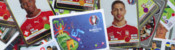Euro 2016 stickers

Comments in green were written by me. Comments in blue were not written by me.

Allowed HTML tags: <br> <a> <small> <b> <i> <s> <sup> <sub> <u> <spoiler> <ul> <ol> <li>
To prove you are not a spam bot, please type "ratio" in the box below (case sensitive):
2015-10-08
This post also appeared on the Chalkdust Magazine blog. You can read the excellent second issue of Chalkdust here, including the £100 prize crossnumber which I set.
From today, the National Lottery's Lotto draw has 59 balls instead of 49. You may be thinking that this means there is now much less chance of winning. You would be right, except the prizes are also changing.
Camelot, who run the lottery, are saying that you are now "more likely to win a prize" and "more likely to become a millionaire". But what do these changes actually mean?

The changes

Until yesterday, Lotto had 49 balls. From today, there are 59 balls. Each ticket still has six numbers on it and six numbers, plus a bonus ball, are still chosen by the lottery machine. The old prizes were as follows:
 Requirement Estimated Prize Match all 6 normal balls £2,000,000 Match 5 normal balls and the bonus ball £50,000 Match 5 normal balls £1,000 Match 4 normal balls £100 Match 3 normal balls £25 50 randomly picked tickets £20,000
The prizes have changed to:
 Requirement Estimated Prize Match all 6 normal balls £2,000,000 Match 5 normal balls and the bonus ball £50,000 Match 5 normal balls £1,000 Match 4 normal balls £100 Match 3 normal balls £25 Match 2 normal balls Free lucky dip entry in next Lotto draw One randomly picked ticket £1,000,000 20 other randomly picked tickets £20,000

Probability of Winning a Prize

The probability of winning each of these prizes can be calculated. For example, the probability of matching all 6 balls in the new lotto is $$\mathbb{P}(\mathrm{matching\ ball\ 1})\times \mathbb{P}(\mathrm{matching\ ball\ 2})\times...\times\mathbb{P}(\mathrm{matching\ ball\ 6})$$ $$=\frac{6}{59}\times\frac{5}{58}\times\frac{4}{57}\times\frac{3}{56}\times\frac{2}{55}\times\frac{1}{54}$$ $$=\frac{1}{45057474},$$ and the probability of matching 4 balls in the new lotto is $$(\mathrm{number\ of\ different\ ways\ of\ picking\ four\ balls\ out\ of\ six})\times\mathbb{P}(\mathrm{matching\ ball\ 1})\times\\...\times\mathbb{P}(\mathrm{matching\ ball\ 4})\times\mathbb{P}(\mathrm{not\ matching\ ball\ 5})\times\mathbb{P}(\mathrm{not\ matching\ ball\ 6})$$ $$=15\times\frac{6}{59}\times\frac{5}{58}\times\frac{4}{57}\times\frac{3}{56}\times\frac{53}{55}\times\frac{52}{54}$$ $$=\frac{3445}{7509579}.$$ In the second calculation, it is important to include the probabilities of not matching the other balls to prevent double counting the cases when more than 4 balls are matched.
Calculating a probability for every prize and then adding them up gives the probability of winning a prize. In the old draw, the probability of winning a prize was $$0.0186$$. In the new draw, it is $$0.1083$$. So Camelot are correct in claiming that you are now more likely to win a prize.
But not all prizes are equal: these probabilities do not take into account the values of the prizes. To analyse the actual winnings, we're going to have to look at the expected amount of money you will win. But first, let's look at Camelot's other claim: that under the new rules you are more likely to become a millionaire.

Probability of winning £1,000,000

In the old draw, the only way to win a million pounds was to match all six balls. The probability of this happening was $$0.00000007151$$ or $$7.151\times 10^{-8}$$.
In the new lottery, a million pounds can be won either by matching all six balls or by winning the millionaire raffle. This will lead to different probabilities of winning on Wednesdays and Saturdays due to different numbers of people buying tickets. Based on expected sales of 16.5 million tickets on Saturdays and 8.5 million tickets on Wednesdays, the chances of becoming a millionaire on a Wednesday or Saturday are $$0.0000001398$$ ($$1.398\times 10^{-7}$$) and $$0.00000008280$$ ($$8.280\times 10^{-8}$$) respectively.
These are both higher than the probability of winning a million in the old draw, so again Camelot are correct: you are now more likely to become a millionaire...
But the new chances of becoming a millionaire are actually even higher. The probabilities given above are the chances of winning a million in a given draw. But if two balls are matched, you win a lucky dip: you could win a million in the next draw without buying another ticket. We should include this in the probability calculated above, as you are still becoming a millionaire due to the original ticket you bought.
In order to count this, let $$A_W$$ and $$A_S$$ be the probabilities of winning a million in a given draw (as given above) on a Wednesday or a Saturday, let $$B_W$$ and $$B_S$$ be the probabilities of winning a million in this draw or due to future lucky dip tickets on a Wednesday or a Saturday (the values we want to find) and let $$p$$ be the probability of matching two balls. We can write $$B_W=A_W+pB_S$$ and $$B_S=A_S+pB_W$$ since the probability of winning a million is the probability of winning in this draw ($$A$$) plus the probability of winning a lucky dip ticket and winning in the next draw ($$pB$$). Substituting and rearranging, we get $$B_W=\frac{A_W+pA_S}{1-p^2}$$ and $$B_W=\frac{A_S+pA_W}{1-p^2}.$$
Using this (and the values of $$A_S$$ and $$A_W$$ calculated earlier) gives us probabilities of $$0.0000001493$$ ($$1.493\times 10^{-7}$$) and $$0.00000009736$$ ($$9.736\times 10^{-8}$$) of becoming a millionaire on a Wednesday and a Saturday respectively. These are both significantly higher than the probability of becoming a millionaire in the old draw ($$7.151\times 10^{-8}$$).
Camelot's two claims—that you are more likely to win a prize and you are more likely to become a millionaire—are both correct. It sounds like the new lottery is a great deal, but so far we have not taken into account the size of the prizes you will win and have only shown that a very rare event will become slightly less rare. Probably the best way to measure how good a lottery is is by working out the amount of money you should expect to win, so let's now look at that.

Expected prize money

To find the expected prize money, we must multiply the value of each prize by the probability of winning that prize and then add them up, or, in other words,
$$\sum_\mathrm{prizes}\mathrm{value\ of\ prize}\times\mathbb{P}(\mathrm{winning\ prize}).$$
Once this has been calculated, the chance of winning due to a free lucky dip entry must be taken into account as above.
In the old draw, after buying a ticket for £2, you could expect to win 78p or 83p on a Wednesday or Saturday respectively. In the new draw, the expected winnings have changed to 58p and 50p (Wednesday and Saturday respectively). Expressed in this way, it can be seen that although the headline changes look good, the overall value for money of the lottery has significantly decreased.
Looking on the bright side, this does mean that the lottery will make even more money that it can put towards charitable causes: the lottery remains an excellent way to donate your money to worthy charities!

Similar postsWorld Cup stickers 2018, pt. 3World Cup stickers 2018, pt. 2A bad Puzzle for TodayA 20,000-to-1 baby?